# LOG#062. SM(IV): SSBEW&Higgs.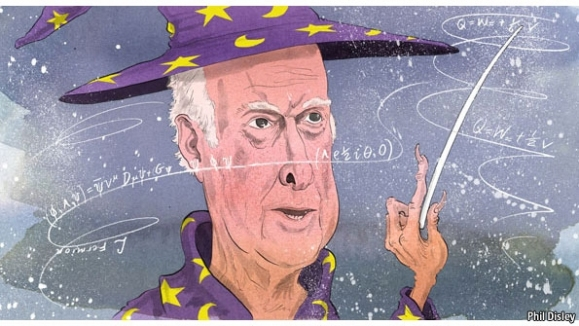We have seen that the SM, under general considerations such as gauge invariance and renormalizability, does NOT initially allow EXPLICIT mass terms in the lagrangian framework for the gauge bosons AND/OR the chiral fermions. Note that every SM fermions is chiral by definition/construction from the beginning.

Remark: MASSLESS gauge bosons are not acceptable in the case of WEAK interactions, which are known to be SHORT-RANGED. In fact, we do KNOW that weak gauge bosons have a non-null mass from high energy experiments ( they were carried out firstly from 1970 to 1980 and extended later till current time in modern colliders).

If the SM, or any other Yang-Mills (YM) theory, describes the Nature in a right way, we must include a mechanism that, respecting the local gauge invariance, allow us at the same time the generation of non zero masses for 3 of the 4 gauge bosons in the EW sector, i.e., we need to invent some “mechanism” that applied to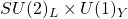will give us the masses of the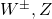bosons, while the photon will remain massless somehow naturally. Moreover, that mechanism will be also inert to the QCD gauge sector, and so, the gluons will be massless too!

A solution was proposed by Glashow, Salam and Weinberg in 1967. They suggested that the gauge invariance of the theory could be “spontaneously broken”. This spontaneous symmetry breaking (SSB) ensures the renormalizability of the theory, and it is based on some pretty nice ideas:

1st. The lowest energy state, called VACUUM in QFT, does NOT respect the gauge symmetry group and induces effective masses for particles propagating through it.

2nd. The fact that the vacuum invariance is not the same thing that the group invariance forces us to introduce some extra fields, called Goldstone fields/bosons. After SSB, some extra degrees of freedom are got by the gauge bosons, and, as Peter Higgs and other remarked in 1964-1965, some degrees of freedom remain after SSB in scalar particles called generically Higgs particles/bosons.

## The SSB and the Higgs mechanism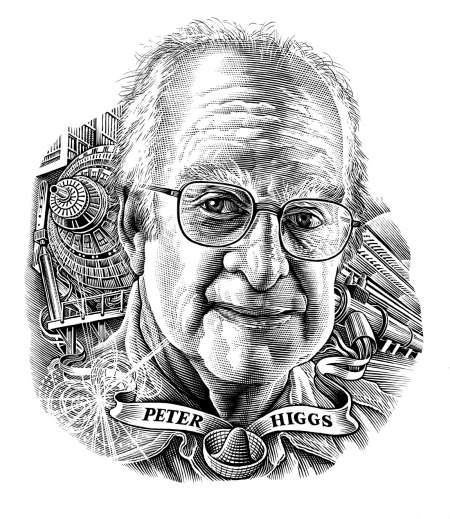The spontaneous symmetry breaking suggested by Glashow, Salam and Weinberg (the so-called GSW model/theory for short) consists in the following prescriptions:

1) Introduce a scalar, complex, doublet field, the so called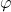Higgs doublet field.

2) Introduce in the SM lagrangian a new extra term for this scalar doublet field: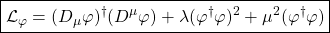3) Due to the potential V in the previous lagrangian, the vacuum expectation value (sometimes called v.e.v. for short) of the complex scalar fields are non null! That is, we getThis fact implies that the lowest energy solution of the classical field theory equations of motion, or ground state/vacuum state, should be a constant, and thus, any nonzero space or time dependencewould increase the energy of the solution. Moreover, one should take the ground state of any other fermion or boson in the SM to have null v.e.v., i.e., for any other SM field not being the Higgs field, we should obtain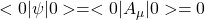since any non-zero v.e.v. for any non-scalar field would violate Lorentz invariance! It is obvious, isn’t it?

The scalar field doublet, a complex field in the SM, can be written in terms of a hermitian basis as a two-component complex field, i.e., a 4-component field with basis elements:and whererepresent (for j=1,2,3,4) four hermitian fields. In the new bais above, the Higgs potential is explicitly rewritten in a very elegant way: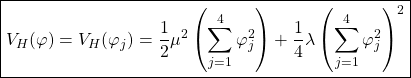This potential is clearly invariant under the orthogonal group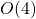. Without loss of generality, we can choose an arbitrary axis in this 4d-field space in such a way that:

1st.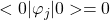but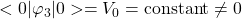.

2nd.This last potential must be MINIMIZED with respect toin order to obtatin the ground state at the classical level ( and the correct equations of motion). We can distinguish two important and different cases:

A). The minimum is located at. The vacuum is the “empty space” and the gauge group ( here,) is unbroken at the mininum.

B)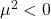. The “critical point”is unstable, and the minium occurs indeed at some nonzero value of. That v.e.v. breaks the gauge symmetryspontaneously. In fact, in our current SM context, the EW point where SSB happens is given by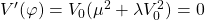and it provides at the minimu a critical value ( v.e.v.)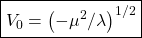The dividing point where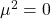can NOT be treated classically. It is necessary to consider the so called “quantum one loop corrections” to the Higgs potential to approach it. If it is the case, then the symmetry will be spontaneously broken.

Then, SSB corresponds to the B) case above, with, and where the Higgs doublet becomes, in first approximation, the classical value: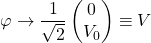Indeed, there is a fun and nice picture showing the idea behind the Higgs potential, also called the “mexican hat” potential: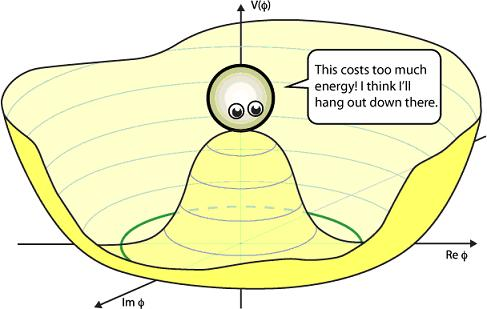There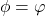and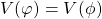is the Higgs potential. The particle is at a maximum (or false vacuum, unstable) and SSB happens when it rolls down to the green circunference, where a non-zero potential is given. It is just a sort of clever trick. In fact, some authors prefer in the name “hidden symmetry” instead of SSB, since, at last, the lagrangian is the same but it is only written with a different set of “more physical” variables.

Let us consider the action of the group generators over this particularly important vacuum state. The generators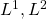of the gauge symmetry group, and the comination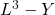are spontaneously broken. It means that: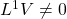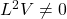By the other hand, the combination of generators Q act like:and thus, the vacuum carries no electric charge in the SM! The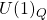subgroup of, the usual electromagnetism, is not spontaneouly broken. Therefore, the symmetry group of the EW interactions,, is spontanously broken down following the next trivial scheme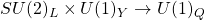To quantize our theory around the classical vacuum, we can shift the scalar field in the next way:where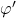are quantum fields with zero v.e.v. To display the physical particle content it is useful to rewrite the four hermitian components ofin terms of a new set of field variables unting the so called “Kibble” parametrization ( it uses a SU(2) gauge transformation). The scalar field can be written in the following form: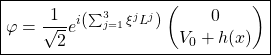and where we have defined four “new” and “auxiliary” fields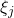and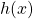. In fact, it shows that the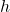field turn out to be the physical Higgs scalar field while the remaining 3 fields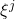disappear from the physical spectrum. SSB broken global symmetries haveas the massless pseudoscalar Goldstone bosons that are necesarrily associated with broke symmetry generators. To prove our assertion about thefield, we must remember that the Kibble parametrizationis nothing else but a SU(2) gauge transformation. The theory, i.e., Nature or the “physical world”, should be invariant under these transformations. Or, explicitly, we can use some gadget named “unitary gauge” if we write: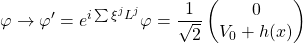Then, the scalar covariant derivative picks a kinetic energy term in the next simple way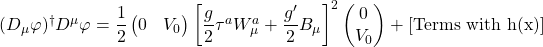and we observe that thebosons are absent, as we wanted to prove. Expanding this last equation, we get the terms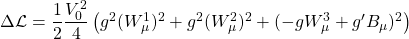Now, we can define the mixing of gauge fields, that is, the “physical” fields: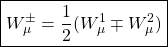Plugging these physical fields into the lagrangian, we obtain the termsand where we have omitted tthe kinetic energy and gauge interaction terms for simplicity. The electrical charge of the physical particlesand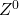is respectivelywhile their masses can be easily read of from the lagrangian terms after the SSB procedure:Furthermore, the remaining combination or mixing of gauge fields, orthogonal to the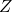field, with it shows to be chargeless and massless, is of course the usual photon field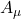:Then, there are two neutral lineal combinations/mixings of fields: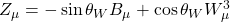or equivalently, in matrix notation,This rotation, lineal combination or mixing with angle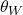, called Weinberg angle, provides a relation between the angle and the coupling constants: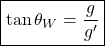Finally, in terms of the Weinberg angle, the Z-boson mass can be written as follows:The SSB mechanism and the Higgs field generation of masses are due to the fact that the W and Z bosons interact continuously with the “condensate” of scalar fields given by the Higgs field and therefore they acquire masses in analogy with a photon propagation through a plasma getting an “effective” mass. The Goldstone fields has disappeared from our theory but they are hidden indeed as the longitudinal degree of freedom of a massive vector particle, plus a Higgs particle.

In summary, after SSB, the Higgs potential becomesWith this trick we have just learned, the 3rd and 4th terms in the rewritten Higgs potential (after SSB) represent the cubic and quartic interactions fo the Higgs scalar field. The second term is a tree-level massThe weak scale is given bywhere we have writtenand the Fermi constant isand thus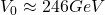The quartic Higgs coupling, the self-interaction of the Higgs field, is unknown. Then, the Higgs mass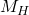is not predicted by the SM and we have to search for it in HEP experiments. A priori, the self-coupling Higgs coupling constant could be any value in the range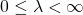Experimentally,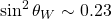as well.

## The fermion masses in the EW-SSB theory

We remarked before that the SM lagrangian does NOT include mass terms in the fermion sector from the beginning. That is, quadratic terms in the fermion fields are not allowed by gauge invariance. One of the most beautiful features of the SSB and the Higgs mechanism is that they can also handle the fermion masses. The fermion mass terms are generated by the introduction of new coupling terms, i.e., extra Yukawa pieces, amaong the leptons, the quarks and the own Higgs field. These coupling are both, gauge invariant and renormalizable from the inner structure of the theory. In fact, these Yukawa fields are the most simple terms with the mentioned characteristics.

We will proceed inductively. Firstly, we can write for the leptons, after diagonalizing the Yukawa matricesSupposing there is a non-zero v.e.v. for the Higgs field, this Yukawa piece introduces a mass term for the electron fieldThe physical mass for the electron field can be read off from this to be: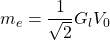Similarly, we can generate masses for any other lepton and the quarks. In the quark case, we get, for instance:from which we would obtain masses for the up and down quarks and whereis the conjugate field of the Higgs, with a net charge under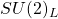and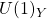equal to zero.

Quarks and leptons from different families/generations have identical weak quantum numbers. It implies that it is possible to include cross Yukawa couplings between interactions. The consequence is that the color eigenstates are NOT the same as the weak eigenstates. These two sets of states stated above ( color and weak eigenstates) are realted by an unitary matrix. In the case of quarks, the matrix is called Cabibbo-Kobayashi-Maskawa (or CKM) matrix. In the case of leptons, the matrix is named Pontecorvo-Maki-Nakagawa-Sakata (or PMNS) matrix. The components of these two matrices, directly and intimately related to the Yukawa couplings, are NOT predicted by the SM. Their values are parameters of the whole theory and they have to be calculated experimentally.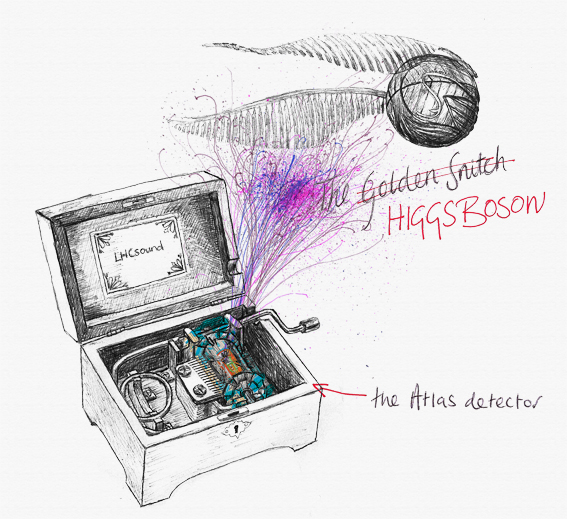This site uses Akismet to reduce spam. Learn how your comment data is processed.